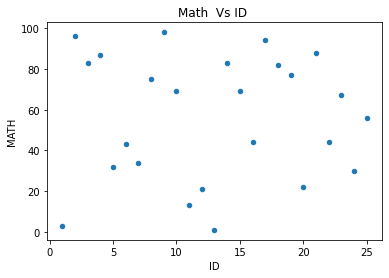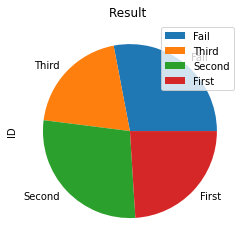# Pandas DataFrame Exercise 1-1

Pandas

## Part I : Creating and grouping data

Create one student mark list with two subjects for 10 ( variable n ) number of students. Marks are given against two subjects and it can vary from 0 to 100. Use random numbers for generating marks. Your DataFrame should have two subject columns Math and Eng. One more column student ID should start from 1 and continue for all students.
Add one more column my_result based on mark in the subject Math. Result ( my_result ) to be arranged in the range 0– 39 Fail, 40 – 50 Third, 50 – 75 Second, 75 to 100 First.

Note that if a student gets 40 then he is passed, If he gets 75 then he is to be placed at First division. ( same is to be followed for other groups )

## Part II ( Data Visualization)

Based on the marks in Math subject plot a scatter graph to show distribution of marks of students.
Create one Pie chart showing the result of total class distributed in bins. Similarly create on Bar chart showing the result.

## Solution

``````import numpy as np
import pandas as pd
n=5 # Number of students , increase this number
my_id=np.arange(1,n+1) # student id from 1 to n

my_math=np.random.randint(0,100,size=n) # 0 to 100 random mark
my_english=np.random.randint(0,100,size=n)

my_pd=pd.DataFrame(data=[my_id,my_math,my_english]).T # transpose the matrix

my_pd.columns=['ID','MATH','ENG'] # adding columns to DataFrame
#print(my_pd.to_string(index=None))

my_labels=['Fail','Third','Second','First'] # labels
my_pd['my_result'] = pd.cut(x=my_pd['MATH'],
bins=[0,40, 50, 75, 100],
labels=my_labels,right=False)
print(my_pd)``````
Output ( Output will change as we are using random numbers as data (mark))
``````   ID  MATH  ENG my_result
0   1    98   54     First
1   2    25   29      Fail
2   3    72   25    Second
3   4    26    7      Fail
4   5    78   79     First``````
Increase the number of students by changing `n=25` to get better distribution of data.

## Data visualization

To the above code we will add for plotting of graphs.
``````my_pd.plot.scatter(title='Math  Vs ID ',x='ID',y='MATH')

my_data=my_pd.groupby(['my_result'])[['ID']].count()
print(my_data)
my_data.plot.pie(title="Result ",y='ID',figsize=(4,4))
my_data.plot.bar(title="Result ",y='ID',figsize=(4,4))``````Subscribe to our YouTube Channel here

## Subscribe

* indicates required
Subscribe to plus2netplus2net.com